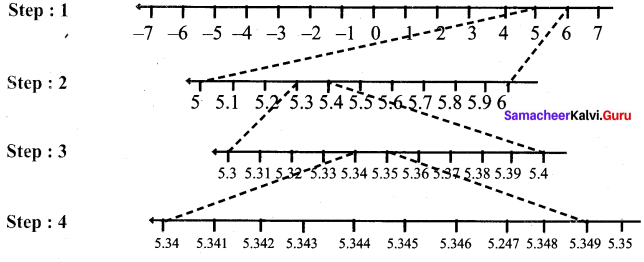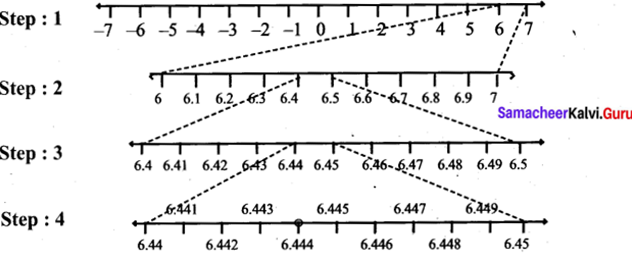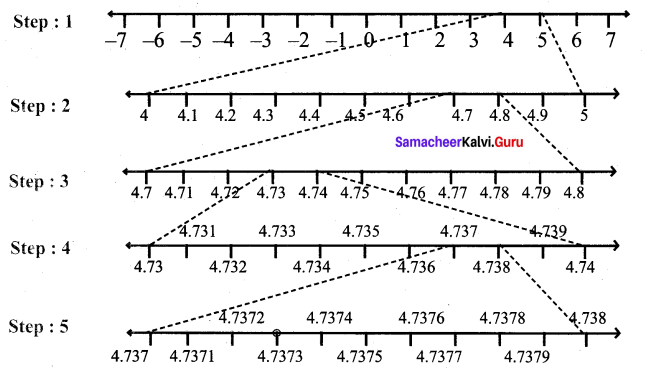# Samacheer Kalvi 9th Maths Solutions Chapter 2 Real Numbers Ex 2.4

## Tamilnadu Samacheer Kalvi 9th Maths Solutions Chapter 2 Real Numbers Ex 2.4

Question 1.
Represent the following numbers on the number line.
(i) 5.348
(ii) $$6.\overline { 4 }$$ upto 3 decimal places.
(iii) $$4.\overline { 73 }$$ upto 4 decimal places.
Solution:
(i) 5.348Step 1 : First we note that 5.348 lies between 5 and 6.
Step 2 : Divide the portion between 5 and 6 into 10 equal parts and use a magnifying glass to visualise that 5.348 lies between 5.3 and 5.4.
Step 3 : Divide the portion between 5.3 and 5.4 into 10 equal parts and use a magnifying glass to visualise that 5.348 lies between 5.34 and 5.35.
Step 4 : Divide the portion between 5.34 and 5.35 into 10 equal parts and use a magnifying glass to visualise that 5.348 lies between 5.348 and 5.349.
Step 5 : Divide the portion between 5.348 and 5.349 into 10 equal parts and use a magnifying glass to visualise that 5.348 lies between 5.349 and 5.348.
We note that 5,349 is visualised closed to 5.349 than to 5.348.

(ii) $$6.\overline { 4 }$$ upto 3 decimal places.
$$6.\overline { 4 }$$ = 6.444
= 6.444 (upto 3 decimal places).
The number lies between 6 and 7.Step 1 : First we note that 6.444 lies between 6 and 7.
Step 2 : Divide the portion between 6 and 7 into 10 equal parts and use a magnifying glass to visualise that 6.4 lies between 6.4 and 6.5.
Step 3 : Divide the portion between 6.4 and 6.5 into 10 equal parts and use a magnifying glass to visualise that 6.4 lies between 6.44 and 6.45.
Step 4 : Divide the portion between 6.44 and 6.45 into 10 equal parts and use a magnifying glass to visualise that 6.444 lies between 6.445 and 6.444. We note that $$6.\overline { 4 }$$ is visualised closed to 6.444 than to 6.445.

(iii) $$4.\overline { 73 }$$ upto 4 decimal places
$$4.\overline { 73 }$$ = 4.73737373
= 4.7373 (correct 4 decimal places).
The number lies between 4 and 5Step 1 : First we note that $$4.7\overline { 3 }$$ lies between 4 and 5.
Step 2 : Divide the portion between 4 and 5 into 10 equal parts and use a magnifying glass to visualise that $$4.7\overline { 3 }$$ lies between 4.7 and 4.8.
Step 3 : Divide the portion between 4.7 and 4.8 into 10 equal parts and use a magnifying glass to visualise that $$4.7\overline { 3 }$$ lies between 4.73 and 4.74.
Step 4 : Divide the portion between 4.73 and 4.74 into 10 equal parts and use a magnifying glass to visualise that $$4.7\overline { 3 }$$ lies between 4.733 and 4.734.
Step 5 : Divide the portion between 4.733 and 4.734 into 10 equal parts and use a magnifying glass to visualise that $$4.7\overline { 3 }$$ lies between 4.7332 and 4.7334.
We note that $$4.7\overline { 3 }$$ is visualised closed to 4.7332 than to 4.7334